GFG App
Open AppBrowser
Continue

Label is a part of JavaFX package . Label is used to display a short text or an image, it is a non-editable text control. It is useful for displaying text that is required to fit within a specific space, and thus may need to use an ellipsis or truncation to size the string to fit. Labels also are useful in that they can have mnemonics which, if used, will send focus to the Control listed as the target of the labelFor property. Label can only a display of text or image and it cannot get focus.
Constructor for the Label class are

1. Label(): Creates an empty label
2. Label(String t): Creates Label with given text.
3. Label(String t, Node g): Creates a Label with the given text and graphic.

Commonly used methods

Below programs illustrate the use of label:

1. Program 1: This program creates a label indicated by the name b. The progress indicator will be created inside a scene, which in turn will be hosted inside a stage (which is the top level JavaFX container). The function setTitle() is used to provide title to the stage. Then a stack-pane is created, on which addChildren() method is called to attach the label inside the scene, along with the resolution specified by (200, 200) in the code. Finally the show() method is called to display the final results.

## Java

 `// Java program to create a label` `import` `javafx.application.Application;` `import` `javafx.scene.Scene;` `import` `javafx.scene.control.*;` `import` `javafx.scene.layout.*;` `import` `javafx.event.ActionEvent;` `import` `javafx.event.EventHandler;` `import` `javafx.scene.control.Label;` `import` `javafx.stage.Stage;` `public` `class` `label ``extends` `Application {`   `    ``// launch the application` `    ``public` `void` `start(Stage s)` `    ``{` `        ``// set title for the stage` `        ``s.setTitle(``"creating label"``);`   `        ``// create a label` `        ``Label b = ``new` `Label(``"This is a label"``);`   `        ``// create a Stack pane` `        ``StackPane r = ``new` `StackPane();`   `        ``// add password field` `        ``r.getChildren().add(b);`   `        ``// create a scene` `        ``Scene sc = ``new` `Scene(r, ``200``, ``200``);`   `        ``// set the scene` `        ``s.setScene(sc);`   `        ``s.show();` `    ``}`   `    ``public` `static` `void` `main(String args[])` `    ``{` `        ``// launch the application` `        ``launch(args);` `    ``}` `}`

1. Output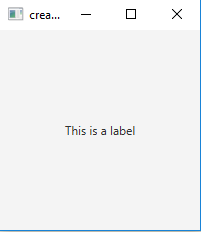1. Java program to create a label with images: This program creates a label with images indicated by the name b, the image is named i and the imageview is indicated by name iw. The label will be created inside a scene, which in turn will be hosted inside a stage (which is the top level JavaFX container). The function setTitle() is used to provide title to the stage. Then a stack-pane is created, on which addChildren() method is called to attach the label with images inside the scene, along with the resolution specified by (200, 200) in the code. Finally the show() method is called to display the final results.

## Java

 `// Java program to create a label with images` `import` `javafx.application.Application;` `import` `javafx.scene.Scene;` `import` `javafx.scene.control.*;` `import` `javafx.scene.layout.*;` `import` `javafx.event.ActionEvent;` `import` `javafx.event.EventHandler;` `import` `javafx.scene.control.Label;` `import` `javafx.stage.Stage;` `import` `javafx.scene.image.*;` `import` `java.io.*;` `public` `class` `label_1 ``extends` `Application {`   `    ``// launch the application` `    ``public` `void` `start(Stage s) ``throws` `Exception` `    ``{` `        ``// set title for the stage` `        ``s.setTitle(``"creating label"``);`   `        ``// create a input stream` `        ``FileInputStream input = ``new` `FileInputStream(``"f:\\gfg.png"``);`   `        ``// create a image` `        ``Image i = ``new` `Image(input);`   `        ``// create a image View` `        ``ImageView iw = ``new` `ImageView(i);`   `        ``// create a label` `        ``Label b = ``new` `Label(``""``, iw);`   `        ``// create a Stack pane` `        ``StackPane r = ``new` `StackPane();`   `        ``// add password field` `        ``r.getChildren().add(b);`   `        ``// create a scene` `        ``Scene sc = ``new` `Scene(r, ``200``, ``200``);`   `        ``// set the scene` `        ``s.setScene(sc);`   `        ``s.show();` `    ``}`   `    ``public` `static` `void` `main(String args[])` `    ``{` `        ``// launch the application` `        ``launch(args);` `    ``}` `}`

1. Output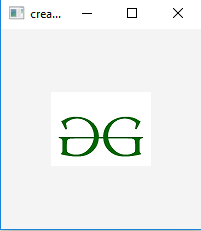1. Java program to create a label with images and text: This program creates a label with images and text indicated by the name b, the image is named i and the imageview is indicated by name iw. The text to be displayed on the label is passed an argument to the constructor of the label. The label will be created inside a scene, which in turn will be hosted inside a stage (which is the top level JavaFX container). The function setTitle() is used to provide title to the stage. Then a stack-pane is created, on which addChildren() method is called to attach the label with images inside the scene, along with the resolution specified by (200, 200) in the code. Finally the show() method is called to display the final results.

## Java

 `// Java program to create a label with images and text` `import` `javafx.application.Application;` `import` `javafx.scene.Scene;` `import` `javafx.scene.control.*;` `import` `javafx.scene.layout.*;` `import` `javafx.event.ActionEvent;` `import` `javafx.event.EventHandler;` `import` `javafx.scene.control.Label;` `import` `javafx.stage.Stage;` `import` `javafx.scene.image.*;` `import` `java.io.*;` `public` `class` `label_2 ``extends` `Application {`   `    ``// launch the application` `    ``public` `void` `start(Stage s) ``throws` `Exception` `    ``{` `        ``// set title for the stage` `        ``s.setTitle(``"creating label"``);`   `        ``// create a input stream` `        ``FileInputStream input = ``new` `FileInputStream(``"f:\\gfg.png"``);`   `        ``// create a image` `        ``Image i = ``new` `Image(input);`   `        ``// create a image View` `        ``ImageView iw = ``new` `ImageView(i);`   `        ``// create a label` `        ``Label b = ``new` `Label(``"Label Text"``, iw);`   `        ``// create a Stack pane` `        ``StackPane r = ``new` `StackPane();`   `        ``// add password field` `        ``r.getChildren().add(b);`   `        ``// create a scene` `        ``Scene sc = ``new` `Scene(r, ``200``, ``200``);`   `        ``// set the scene` `        ``s.setScene(sc);`   `        ``s.show();` `    ``}`   `    ``public` `static` `void` `main(String args[])` `    ``{` `        ``// launch the application` `        ``launch(args);` `    ``}` `}`

1. Output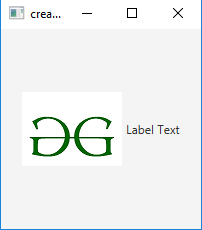1. Java program to create a labels and textfield and use setLabelFor property: In this program the labels are set for mnemonic parsing (if Alt+1 is presses then focus shifts to first textfield and if Alt + 2 is presses then focus shifts to second textfield.

## Java

 `// Java program to create a labels and textfield and use setLabelFor property` `import` `javafx.application.Application;` `import` `javafx.scene.Scene;` `import` `javafx.scene.control.*;` `import` `javafx.scene.layout.*;` `import` `javafx.event.ActionEvent;` `import` `javafx.event.EventHandler;` `import` `javafx.scene.control.Label;` `import` `javafx.stage.Stage;` `import` `javafx.scene.image.*;` `import` `java.io.*;` `public` `class` `label_3 ``extends` `Application {`   `    ``// launch the application` `    ``public` `void` `start(Stage s) ``throws` `Exception` `    ``{` `        ``// set title for the stage` `        ``s.setTitle(``"creating label"``);`   `        ``// TextField` `        ``TextField b1 = ``new` `TextField(``"textfield"``);`   `        ``// create a label` `        ``Label b = ``new` `Label(``"_1 TextField"``);`   `        ``// setlabel for` `        ``b.setLabelFor(b1);`   `        ``// TextField` `        ``TextField b4 = ``new` `TextField(``"textfield"``);`   `        ``// create a label` `        ``Label b3 = ``new` `Label(``"_2 TextField"``);`   `        ``// setlabel for` `        ``b3.setLabelFor(b4);`   `        ``// create a Tile pane` `        ``TilePane r = ``new` `TilePane();`   `        ``// setMnemonic` `        ``b.setMnemonicParsing(``true``);` `        ``b3.setMnemonicParsing(``true``);`   `        ``// add password field` `        ``r.getChildren().add(b);` `        ``r.getChildren().add(b1);` `        ``r.getChildren().add(b3);` `        ``r.getChildren().add(b4);`   `        ``// create a scene` `        ``Scene sc = ``new` `Scene(r, ``200``, ``200``);`   `        ``// set the scene` `        ``s.setScene(sc);`   `        ``s.show();` `    ``}`   `    ``public` `static` `void` `main(String args[])` `    ``{` `        ``// launch the application` `        ``launch(args);` `    ``}` `}`

1. Output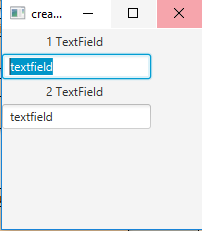1.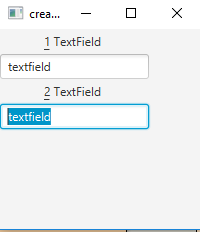Note: The following programs might not run in an online IDE please use an offline compiler. You should have the latest version of Java to run this programs.
Reference: https://docs.oracle.com/javase/8/javafx/api/javafx/scene/control/Label.html

My Personal Notes arrow_drop_up